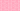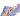PricingResources

# What is the High-Low Method?

Written by

The high-low method is an accounting technique used to separate out fixed and variable costs in a limited set of data.

It involves taking the highest level of activity and the lowest level of activity and comparing the total costs at each level. If the variable cost is a fixed charge per unit and fixed costs remain the same, it is possible to work out the fixed and variable costs by solving the equations.

However, users must be cautious of the high-low method. While it is easy to apply, it can distort costs and yield more or less accurate results because of its reliance on two extreme values from one data set.

## High-low method formula

The high-low method provides a simple way to split fixed and variable components of combined costs using a few formula steps. First you calculate the variable cost component and fixed cost component, then plug the results into the cost model formula.

Once you have the variable cost per unit, you can calculate the fixed cost.

Then use all the results to calculate the high–low cost using this formula:

## High-low method example

Bonnie runs a small car factory in Detroit and needs to know the expected amount of overheads the factory will incur in the next month.

Factory overheads cost for the four months prior were as follows:

 Cost Units January \$60,000 10,000 February \$40,000 7,500 March \$50,000 6,000 April \$55,000 5,500

Bonnie expects to produce 8,000 units in May.

Step 1: Identify the highest and lowest activity level

The highest activity level is 10,000 units in January (highest activity cost is \$60,000)

The lowest activity level is 5,500 units in April (lowest activity cost is \$55,000)

Step 2: Calculate the variable cost per unit

Use the formula shown above to work it out:

Variable cost per unit = (\$60,000 − \$55,000) ÷ (10,000 − 5,500)

Variable cost per unit = \$5,000 ÷ 4,500 = \$1.11 per unit

Step 3: Calculate the fixed cost

Use the formula shown above to work it out:

Fixed cost = \$60,000 − (\$1.11 x 10,00) = \$48,900

Step 4: Calculate the total variable cost for the new activity

Multiply the variable cost per unit (step 2) by the number of units expected to be produced in May to work out the total variable cost for the month.

Total variable cost = \$1.11 x 8,000 = \$8,880

Step 5: Calculate the total cost

Now add the fixed cost (step 3) and variable cost for the new activity (step 4) together to get the total cost of overheads for May.

Total cost = \$48,900 + \$8,880 = \$57,780

## High-low method limitations

The high-low method does not consider small details such as variation in costs. It assumes that fixed and unit variable costs are constant, which is not always the case in real life.

There are also other cost estimation tools that can provide more accurate results. The least-squares regression method takes into consideration all data points and creates an optimized cost estimate. It can be easily and quickly used to yield significantly better estimates than the high-low method.

## We can help

If you’re interested in finding out more about fixed overhead volume variance, then get in touch with the financial experts at GoCardless. Find out how GoCardless can help you with ad hoc payments or recurring payments.## Interested in automating the way you get paid? GoCardless can help

Contact sales

Sales

Contact Sales

+1(415) 523-2279

Support

help@gocardless.com

+1 (628) 241-0044